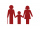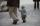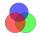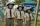# Pupil

I'm a primary school pupil. I attended the parents' exercises with children 1/4 of my age, 1/3 for drawing, and 1/6 for flute. For the first three years of my life, I had no ring, and I never went to two rings simultaneously. How old am I?

x =  12

### Step-by-step explanation:

x/4+x/3+x/6+3=x

3x = 36

x = 12

Our simple equation calculator calculates it.Did you find an error or inaccuracy? Feel free to write us. Thank you!Tips to related online calculators
Need help to calculate sum, simplify or multiply fractions? Try our fraction calculator.
Do you have a linear equation or system of equations and looking for its solution? Or do you have a quadratic equation?
Do you want to convert time units like minutes to seconds?

## Related math problems and questions:

• Pupil ageI am a pupil of the primary school, and I spent 1/4 of my age on parents' exercise with children, 1/3 on drawing, 1/6 on flute. I did not have a ring for the first three years of my life and never went to two rings at once. How old am I?Add marks (+, -, *, /, brackets) to fullfill equations 1 3 6 5 = 10 This is for the 4th grade of the primary school - with no negative numbers yet
• 3 children3 children had to divide 4 pounds is candy. How much candy did each child get?
• Brother and sisterWhen I was 8, my sister was 4, and now I am 18. How old is my sister?
• If BenIf Ben is ⅕ the age of his father, ¼ the age of his mother, and twice the age of his sister, how old are his parents and sister if he is 8 years old?Why does 1 3/4 + 2 9/10 equal 4.65? How do you solve this?
• The fatherThe father is 36 years old. The son is 6 years old. How many years will a father be 3 times older than a son?
• GroupA group of kids wanted to ride. When the children were divided into groups of 3 children, one remain. When divided into groups of 4 children, 1 remains. When divided into groups of 6 children, 1 remains. After divided into groups of 5 children, no one lef
• Rings - intersectThere are 15 pupils on the sporting ring. 10 pupils go to football, 8 pupils go to floorball. How many pupils go to both rings at the same time?Add two mixed fractions: 2 4/6 + 1 3/6
• FractionsSort fractions z1 = (6)/(11); z2 = (10)/(21); z3 = (19)/(22) by its size. Result write as three serial numbers 1,2,3.
• Sum three fractionsWork out the sum of 1/4, 1/5 and 3/10.
• Age problemsA) Alex is 3 times as old as he was 2 years ago. How old is he now? b) Casey was twice as old as his sister 3 years ago. Now he is 5 years older than his sister. How old is Casey? c) Jessica is 4 years younger than Jennifer now. In 10 years, Jessica will
• Fractions and mixed numerals(a) Convert the following mixed numbers to improper fractions. i. 3 5/8 ii. 7 7/6 (b) Convert the following improper fraction to a mixed number. i. 13/4 ii. 78/5 (c) Simplify these fractions to their lowest terms. i. 36/42 ii. 27/45 2. evaluate the follow
• Cakes1/3 poppy cake, 1/3 apple, 15 pieces of cheese. How many are totally cakes?
• CampIn the camp are children. 1/2 went on a trip, 1/4 went to bathe, and 38 children remained in the room. How many children are in the camp?
• The boyThe boy scouts spent 10/12 hour doing their daily exercises. They only used 1/4 hour in hiking. How much time did they use for other body exercises?